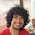### Sink the Submarine

Source: http://www.ocf.berkeley.edu/~wwu/riddles

Problem:
An enemy submarine is somewhere on the number line (consider only integers for this problem). It is moving at some rate (again, integral units per minute). You know neither its position nor its velocity.

You can launch a torpedo each minute at any integer on the number line. If the the submarine is there, you hit it and it sinks. You have all the time and torpedoes you want. You must sink this enemy sub - devise a strategy that is guaranteed to eventually hit the enemy sub.

A similar problem we solved some time back was "Finding a Hermit"

Solution:
Posted by Aaditya Ramdas (CSE, IITB Alumnus working at Tower Research) in comments!!

1.well I was asked this question in my morgan stanley interview.. didnt get it without a hint. so well im not posting the ans coz its so good!

2.It is good indeed. :)

3.ok, i have never seen this problem before, it looked very interesting at first, but in a few mins it seemed rather normal :P

We don't know the initial position I, velocity V. We know that at time t, he is at I+vt.

So essentially, if we think of his initial position as a point on the plane formed by IxV, we just have to find it sequentially. So, we do it in an outwardly increasing squares search.

So at t=0, guess his position I+vt as if his (I,v) was (0,0). Then at t=1, guess his position I+vt as if his (I,v) was (0,1). Then (1,0), (0,-1), (-1,0), then move to the outer square of (1,1) to (-1,-1) and so on.

Ultimately we have to hit (I,v) so we will catch him at that time. Infact it is pretty easy to calculate the exact time at which you will catch (I,v) - it will be while doing the square covering the points abs(I)+abs(v)...

It's very similar to proving that rationals are countable.

And also, it is easy to see that if this problem is generalised to I,v,acceleration a, etc it doesnt matter, solution is simply extendable...

4.@Ramdas.. correct solution (as usual :P) ..
Thanx

BTW, Nice observation regarding acceleration :)

5.can anyone please give a proof that this solution will work

6.:-o @Ashu..
The problem is effectively to find values of I and V.

So, check for all values of I and V. Since the set NXN is countable set {Also used in proving that set of rational numbers is countable}, we can check for I + VT in countable time and hence get the ship.

Does that clarify?

In case you don't know what countably infinite set is, read this wikipedia article.

7.This comment has been removed by the author.

8.Well, the above solution is correct. But. I have a different view. Maybe it's not correct.

Since, I have been given the freedom of using any amount of torpedoes. I can use this fact in my arguments.
Suppose I am at position 0 at t = 0 on the number line. The initial enemy's position can be odd or even and the magnitude of velocity can also be odd or even,
So, first at t = 0, I launch at all odd positions. If I hit submarine, its good. Else, now I launch torpedoes at every even positions. If I hit, its good. Else now I again launch torpedoes at every even positions. This time I will certainly hit the submarine.
The reason being if it is not at odd at t = 0. Then , it is at even, If I do not find the subamrine at even positions the second time, then it is moving with odd |velocity|. This means the third time it will be at even.
Hope this is correct, given the constraints :-P

1.Consider any one one function from f: Z X Z to N.(it is possible as both are countable). Now if (a,b) is the pair corresponding to initial position and velocity of submarine, it is destroyed at time f((a,b))